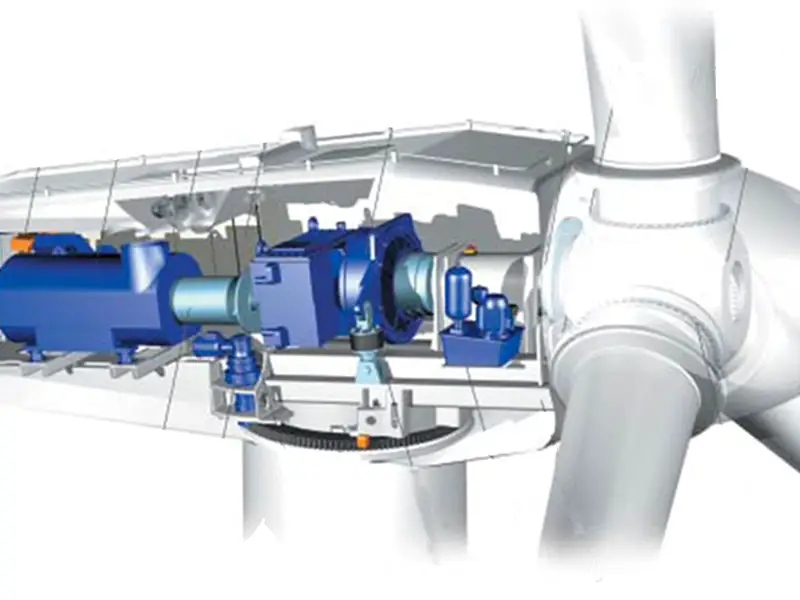Modern large-scale grid-connected wind turbines above the megawatt level mostly use wind turbine blades that can be adjusted in pitch and operate at variable speeds. As we all know, when the variable speed wind turbine is running, the generator connected to it will also run at a variable speed. Therefore, a generator with constant frequency and constant voltage energy that can be emitted when the variable speed wind turbine is running at a variable speed must be used to realize the connection with the power grid. This goal can be achieved by combining the doubly-fed asynchronous generator with wound rotor with the IGBT inverter and PWM control technology applying the latest power electronic technology, that is, a variable-speed constant-frequency power generation system.

## 1. Variable speed wind turbine drive generator system composition

The following figure shows the connection between the variable-speed constant-frequency power generation system composed of variable-pitch variable-speed wind turbines and doubly-fed asynchronous generators and the power grid. When the wind speed of the variable speed wind turbine changes, the system works as follows: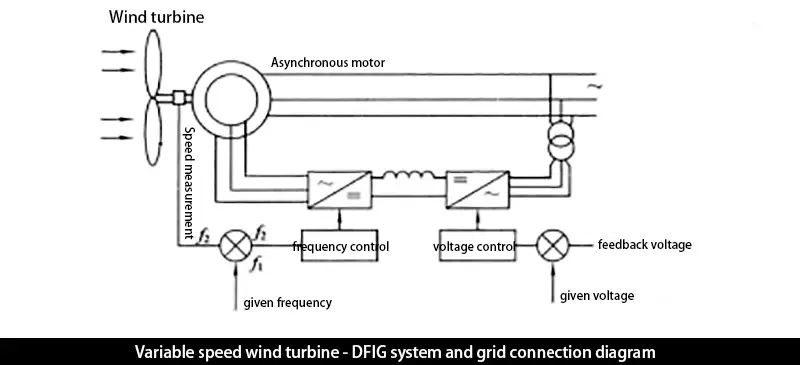When the wind speed of the variable speed wind turbine decreases, the speed of the wind turbine of the variable speed wind turbine decreases, and the rotor speed of the asynchronous generator also decreases, the rotating magnetic field speed generated by the rotor winding current will be lower than the synchronous speed ns of the asynchronous motor, and the frequency f of the induced electromotive force in the stator winding is low. At f1, at the same time, the speed measuring device immediately feeds back the information of the speed reduction to the circuit that controls the frequency of the rotor current to increase the frequency of the rotor current, then the speed of the rotor rotating magnetic field rises back to the synchronous speed ns, so that the frequency of the induced potential of the stator winding is The factory returns to the rated frequency again.

Similarly, when the wind speed of the variable speed wind turbine increases, the rotor speed of the variable speed wind turbine and the asynchronous motor increases, and the frequency of the induced electromotive force of the stator winding of the asynchronous generator will be higher than the frequency f1 corresponding to the synchronous speed, and the speed measuring device will immediately The information of the frequency increase is fed back to the circuit that controls the frequency of the rotor current, so that the frequency of the rotor current is reduced, so that the speed of the rotor rotating magnetic field returns to the synchronous speed ns, and the frequency of the induced electromotive force of the stator winding is restored to the frequency f1. It must be noted that, When running supersynchronously, the direction of rotation of the rotor's rotating magnetic field should be opposite to that of the rotor itself. Therefore, when running supersynchronously, the rotor windings should be able to automatically change the phase sequence to reverse the direction of rotation of the rotor's rotating magnetic field.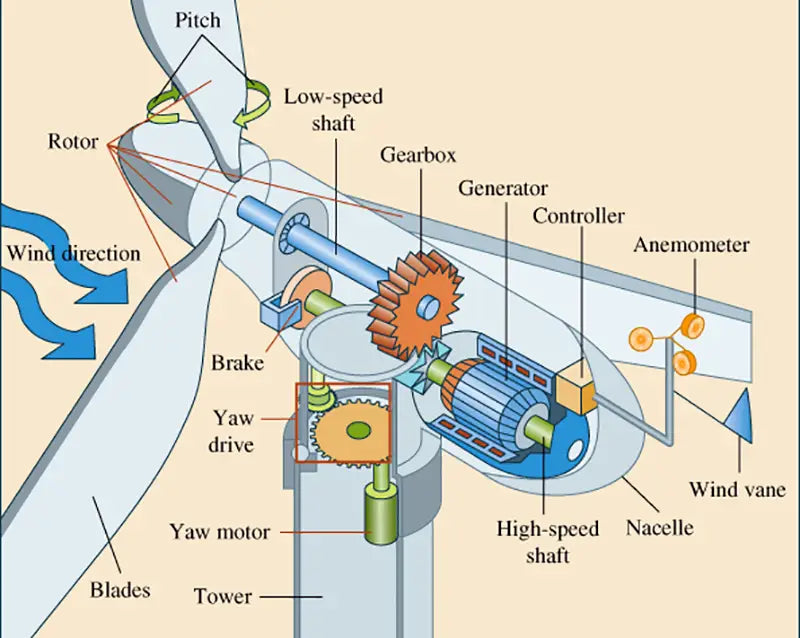When the rotor speed of the asynchronous motor reaches the synchronous speed, the frequency of the rotor current should be zero at this time, that is, the rotor current is a DC current, which is the same as when a DC current is passed into the rotor excitation winding of an ordinary synchronous generator. In fact, in this case The lower double-fed asynchronous generator is already the same as the ordinary synchronous generator.

As shown in the figure above, the control of the output terminal voltage of the doubly-fed asynchronous generator is realized by controlling the rotor current of the generator. When the load of the generator increases, the voltage of the output terminal of the generator decreases. This information is obtained from the voltage detection, and the Feedback to the circuit that controls the size of the rotor current, that is, by controlling the conduction angle of the thyristor of the three-phase half-controlled or fully-controlled rectifier bridge, the conduction angle is increased, so that the rotor current of the generator increases, and the induced electromotive force of the stator winding increases. If it is large, the generator output voltage will return to the rated voltage. Conversely, when the generator load decreases, the voltage at the output terminal of the generator increases, and the feedback information obtained after the voltage detection will reduce the conduction angle of the thyristor of the half-controlled or fully-controlled rectifier bridge, thereby reducing the rotor current. The stator winding output voltage drops back to the rated voltage.

## 2. Variable-speed wind turbine drive generator inverter and control method

In the variable-speed constant-frequency wind power generation system composed of doubly-fed asynchronous generators, different types of cycloconverters can be used as frequency converters in the rotor circuit of the asynchronous generators.

(1) Adopt AC-DC-AC voltage type forced commutation inverter. The use of this type of frequency converter can realize a smooth transition from synchronous operation to super-synchronous operation, which can expand the operating range of the variable-speed wind turbine. In addition, due to the use of forced commutation, the power factor can also be adjusted, but because the rotor current is A square wave, which produces low-order spectral torque in the motor.

(2) The use of AC-AC inverter can save the DC link in the AC-DC-AC inverter, and can realize the smooth transition from sub-synchronous to super-synchronous operation and realize the adjustment of power factor. There are many thyristors, and at the same time, low-order harmonic torque is also generated in the motor.

(3) Frequency converter with pulse width modulation. The IGBT inverter and PWM control technology of the latest power electronic technology can obtain sinusoidal rotor current, no low-order harmonic torque will be generated in the motor, and at the same time, the power factor can be adjusted, and the modern double-fed asynchronous power generation above the megawatt level This kind of inverter is mostly used in the machine.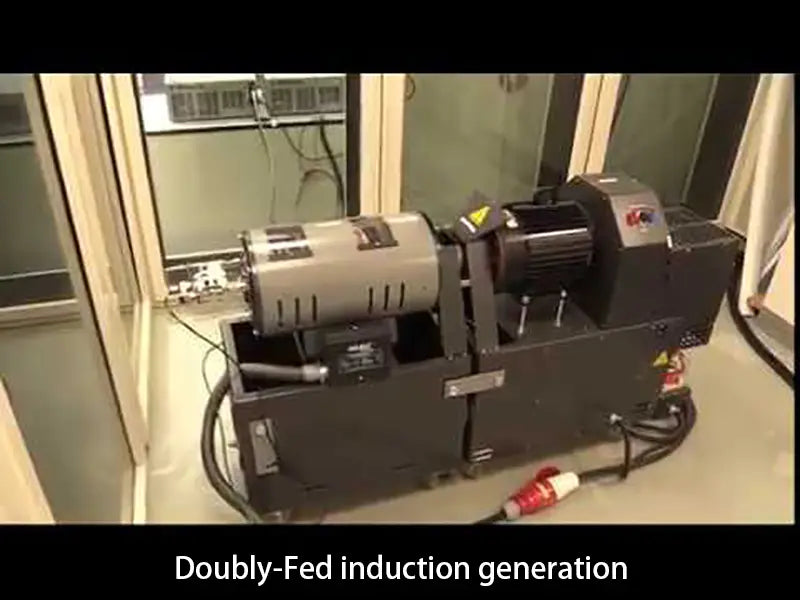## 3. Technical data of megawatt units

The technical data of the medium and large variable speed constant power generation system composed of variable pitch wind turbines and doubly fed asynchronous generators developed abroad are as follows: the maximum variation range of the asynchronous generator slip rate is -25%~35%.

The output active power of asynchronous generator is 300-3000 kw.

Determine the operating data of a 1.5 MW, 4-pole, doubly-fed asynchronous generator as shown in the figure below.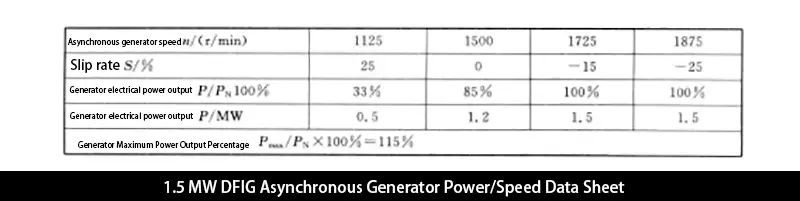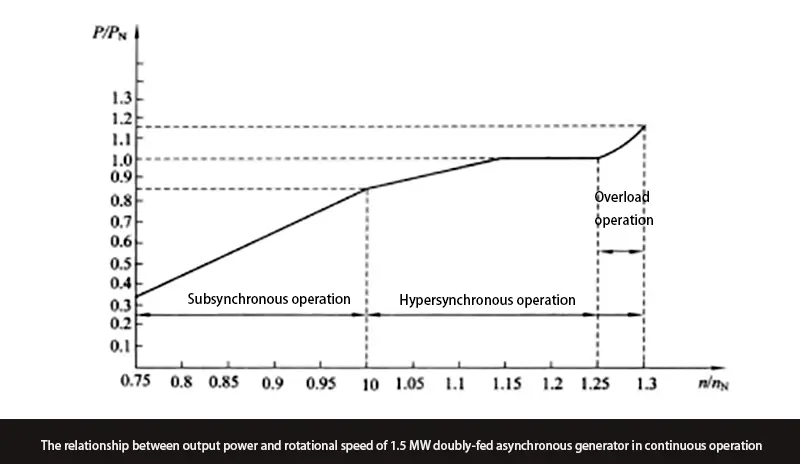## 4. Advantages of variable speed wind turbine system

(1) This variable speed wind turbine has the ability to control the slip of the asynchronous generator to change within an appropriate value range, so it can optimize the pitch adjustment of the wind turbine blades, that is, it can reduce the number of wind turbine blade pitch adjustments, This is advantageous for the pitch adjustment mechanism.

(2) The variable speed wind turbine can reduce the noise level of the wind turbine during operation.

(3) The variable speed wind turbine can reduce the severe torque fluctuation of the unit, thereby reducing the mechanical stress of all components, which provides a strong guarantee for reducing the quality of components or developing large-scale wind turbines

(4) Since it is a variable-speed wind turbine, the operating speed of the variable-speed wind turbine can be adjusted to the optimal efficiency value of the wind turbine in a wide range, so that the Cp value of the variable-speed wind turbine can be optimized, so as to obtain high system efficiency. .

(5) It can realize the low-fluctuation and smooth electric power output of the generator, optimize the power grid quality in the system, and reduce the temperature change of the generator at the same time.

(6) The variable speed wind turbine is simple to connect with the power grid, and can realize the adjustment of power factor.

(7) Independent operation can be achieved, and several identical operating units can also be operated in parallel.

(8) The content of the transformer in this variable speed constant frequency system depends on the maximum slip power of the generator during variable speed operation. Generally, the maximum slip rate of the motor is (-25~+35)%, so the maximum slip of the inverter is The capacity is only 1/4~1/3 of the rated capacity of the generator.